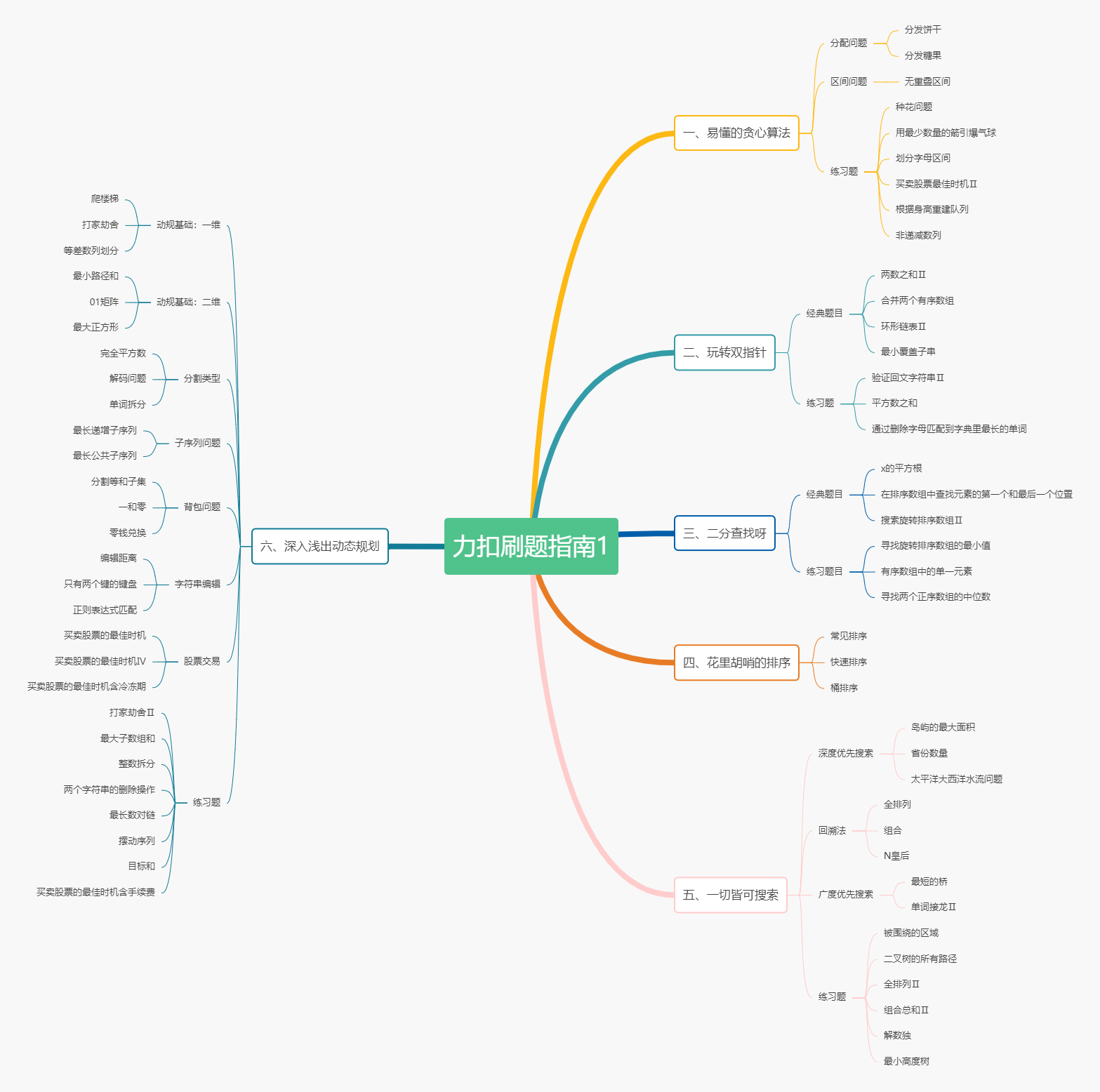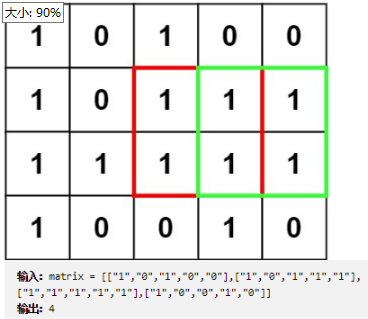# 【力扣刷题笔记】由简到难，模块突破， 你与AC只差一句提示

anan1周前 (05-10)后端29# 二、玩转双指针

## 经典题目

### 88.合并两个有序数组

merge(int[] nums1, int m, int[] nums2, int n)， 给两个有序数组，和长度m、n，把数组合并到数组1

# 三、二分查找

## 练习题目

### 154.寻找旋转排序数组的最小值

nums[mid] < nums[r] 则右边一定是排序区间（找左边）
nums[mid] > nums[r] 则 左边一定是排序区间找右边（如果右边也是排序的，那最小值就在mid+1，所以还是找右边区间）

# 五、一切皆可搜索

## 深度优先搜索

### 547.省份数量

DFS+一层遍历，标志位为一维城市数组，而不是关系图。

### 417.太平洋大西洋水流问题

DFS水往高处流，先从左上流上去，然后做标记，再从右下流上去，根据标记判断返回

## 回溯

### 77.组合

DFS，每个数选或者不选，先选试试，返回结果后就不选这个位置往后走。注意剩余长度和路径不够组成新的组合的时候，需要返回，cur无限增大。

### 51.N皇后

n 皇后问题 研究的是如何将 n 个皇后放置在 n×n 的棋盘上，并且使皇后彼此之间不能相互攻击。（不在同一行同一列同一斜线）

# 六、深入浅出动态规划

## 动态规划基础：一维

### 70.爬楼梯

n阶楼梯， 每次可以走一步或者两步， 求走到n阶有多少种方式。

## 动态规划基础：二维

### 64.最小路径和

dp[ i ][ j ] 表示到 一个位置的最小路径和 ，可以用给grid矩阵代替dp数组grid[ i ][ j ] += min(grid[i - 1][ j ], grid[ i ][j - 1])

### 221.最大正方形## 分割类型

### 279.完全平方数

dp[ i ] 表示数字 i 最少可以由几个完全平方数构成， 很明显 dp[ i ] 和 dp[ i - 1]、 dp[ i - 4 ]、dp[ i - 9 ]等相关。

### 139.单词拆分

dp[ i ] 表示 s[0-i] 能否分割后都在集合中找到。

## 子序列问题

### 300.最长递增子序列

1.动态规划： dp[ i ] 表示到 i 的最长递增子序列， dp[ i ] = max(dp[ i - j ] + 1 ) (j 是 比 i 小的数的位置)
2.贪心 + 二分 ： 遇到更大的数就加到数组后面， 遇到数组最大数小的数， 就把之前较小的数替换成这个数。
（贪心思想： 每次递增都增较小的值，最后这个数组一定是最长的）

### 1143.最长公共子序列

text1 = “abcde”, text2 = “ace”

## 背包问题

0-1背包： 限定每个物品只能拿 0或1个

### 416.分割等和子集

dp[ i ][ j ]表示到第 i 个数字后， 存在最大和为 j 的子序列。

dp[ i ] 表示最大和为 i 的子序列是否存在。
dp[j] |= dp[j - nums[i]] 当前这个数nums[i]能配合之前的结果组成吗？
j 从大至小，防止重复利用nums[ i ]。 核心代码：

``````  		int n = nums.length, target = sum / 2;
boolean[] dp = new boolean[target + 1];
dp = true;
for(int i = 0; i < n; i++) {
for(int j = target; j >= nums[i]; j--) {
dp[j] |= dp[j - nums[i]];  //当前这个数nums[i]能配合之前的结果组成吗？
//j 从大至小，不会出现重复利用的情况因为在i 没有增大之前，较小的 j 是用不到较大 j 的结果的
}
}
``````

### 474.一和零

dp[ i ][ j ] = max(dp[i][j], dp[i - count][j - count] + 1)
0-1背包，压缩，最外层是给的选择，内层是条件 i 、 j，从大到小，防止重复。 核心代码

``````        for(String s : strs) {
int[] count = count(s);
for(int i = m; i >= count; i--) {
for(int j = n; j>= count; j--) {
dp[i][j] = Math.max(dp[i][j], dp[i - count][j - count] + 1);
}
}
}
``````

### 322.零钱兑换

``````        for(int i = 1; i <= amount; i++) {
for(int coin : coins) {
if(i >= coin) {
dp[i] = Math.min(dp[i], dp[i - coin] + 1);
}
}
}
``````

## 字符串编辑

（删除和插入是一样的）

### 650.只有两个键的键盘

dp[ i ] 表示 i 个 A 需要的最少操作次数

``````        for(int i = 2; i < n + 1; i++) {
dp[i] = i;
for(int j = 2; j <= Math.sqrt(i); j++) {
if( i % j == 0) {
dp[i] = dp[j] + dp[ i / j];
}
}
}
``````

### 10.正则表达式匹配

’ . ’ ， 能匹配任意字符
‘ * ’， 能匹配任意数量的前一个字符

``````        boolean[][] dp = new boolean[m + 1][n + 1];
//把所有*取0个，初始化dp
dp = true;
for(int i = 1; i < n + 1; i++) {
if(p.charAt(i - 1) == '*') {
dp[i] = dp[i - 2];
}
}
for(int i = 1; i <= m; i++) {
for(int j = 1; j <= n; j++) {
if(p.charAt( j - 1) == '.') {
//任意字符
dp[i][j] = dp[i - 1][j - 1];
}else if(p.charAt(j - 1) != '*') {
//字母
dp[i][j] = dp[i - 1][j - 1] && s.charAt(i - 1) == p.charAt(j - 1) ;
}else if(p.charAt(j - 2) != '.' && p.charAt(j - 2) != s.charAt(i - 1)) {
// 当前是 * , 且前一个不符合, *只能是取0个了
dp[i][j] = dp[i][j - 2];
}else {
// 当前是 * , 且前一个符合, 取0个（删掉表达式*和前一个字母）， 取1个（删掉表达式*）, 取n个（删掉字母）
dp[i][j] = dp[i][j - 2] || dp[i][j- 1] || dp[i - 1][j];
}
}
}
``````

## 股票交易

### 188.买卖股票最佳时机Ⅳ

``````        //初始化
for(int i = 0; i <= k; i++) {
}

for(int i = 0; i < n; i++) {
for(int j = 1; j <= k; j++) {
sell[j] = Math.max(sell[j], buy[j] + prices[i]);
}
}
``````

### 309.最佳买卖股票时机含冷冻期``````        buy = -prices; //初始化
s1 = -prices; // 初始化
for(int i = 1; i < n; i++) {
buy[i] = s2[i - 1] - prices[i];
s1[i] = Math.max(s1[i - 1], buy[i - 1]);
sell[i] = Math.max(s1[i - 1], buy[i - 1]) + prices[i];
s2[i] = Math.max(s2[i - 1], sell[i - 1]);
}
``````

## 练习题

### 343.整数拆分

dp[ i ] 表示 数字 i 拆分后最大乘积。

``````dp[i] = max(dp[i], j * (i - j), j * dp[i - j])
``````

## 进阶练习

### 646.最长数对链

① 动态规划，dp[ i ] 表示以 数对 i 为结尾的最长数对链。 需要先对数组进行排序，使尾小的一定先得到结果。
②贪心。 贪心思想：每次递增最小，最后得到最长递增。

### 376.摆动序列

``````        down = up = 1;
for(int i = 1; i < n; i++) {
//不需要遍历之前的数,因为摆动子序列长度是一直不减的。 就算出现连续递增，只要有下降沿，down[i] 就能增加一个，和之前无关最后递增的数无关
if(nums[i] > nums[i - 1]) {
up[i] = down[i - 1] + 1;
down[i] = down[i - 1];
}else if(nums[i] < nums[i - 1]) {
down[i] = up[i - 1] + 1;
up[i] = up[i - 1];
}else {
up[i] = up[i - 1];
down[i] = down[i - 1];
}
}
``````

### 494.目标和

``````    public int findTargetSumWays(int[] nums, int target) {
int sum = 0;
for(int num : nums) sum += num;
if((target + sum) % 2 != 0) return 0;
int x = (target + sum) / 2;
if(x < 0) return 0; // 如果x为负值，说明取不到
int[] dp = new int[x + 1];
dp = 1;
for(int num : nums) {
for(int j = dp.length - 1; j >= num; j--) {
dp[j] += dp[j - num];
}
}
return dp[x];
}
``````

### 714.买卖股票的最佳时机含手续费``````        buy = s1 = -prices;
for(int i = 1; i < n; i++) {
buy[i] = Math.max(s2[i - 1], sell[i - 1]) - prices[i];
s2[i] = Math.max(sell[i - 1], s2[i - 1]);
sell[i] = Math.max(s1[i - 1], buy[i - 1]) + prices[i] - fee;
s1[i] = Math.max(s1[i - 1], buy[i - 1]);
}
``````

,https://blog.csdn.net/weixin_44179010/article/details/123847312### 史上最全 SpringCloudAlibaba入门教程，从零开始带你深入学习（一）——环境搭建

SpringCloudAlibaba（一）——环境搭建 SpringCloudAlibaba简介 springcloud spring团队开源微服务工具集，帮助我们快速构建分布式系统（微服务系统...

### # Day11-Java基础

Day11-Java 文章目录 Day11-Java 1、Eclipse开发工具...